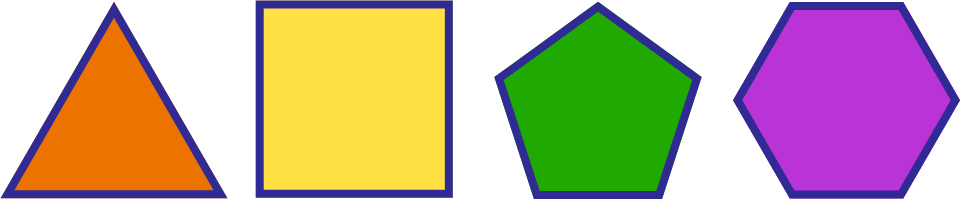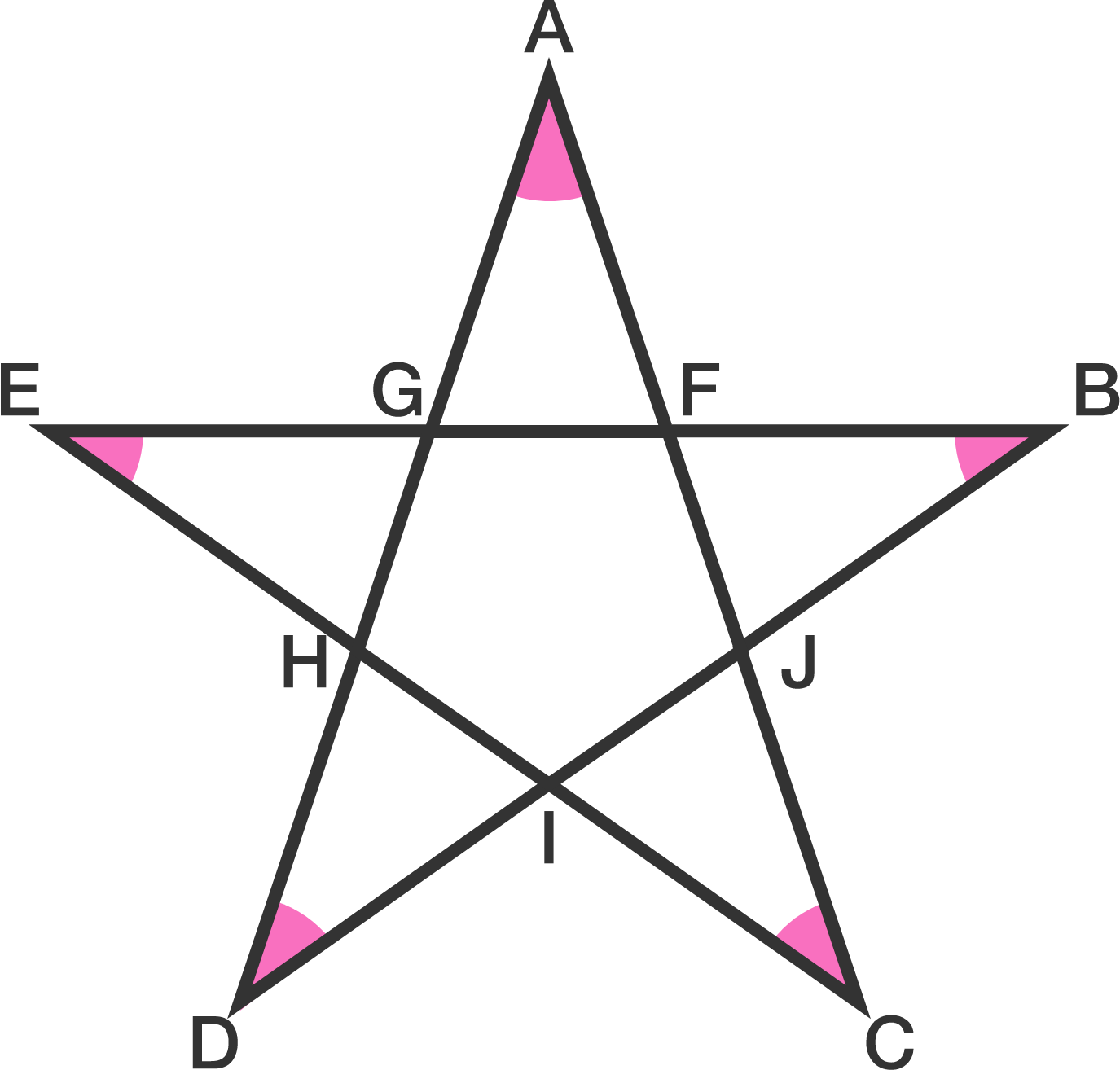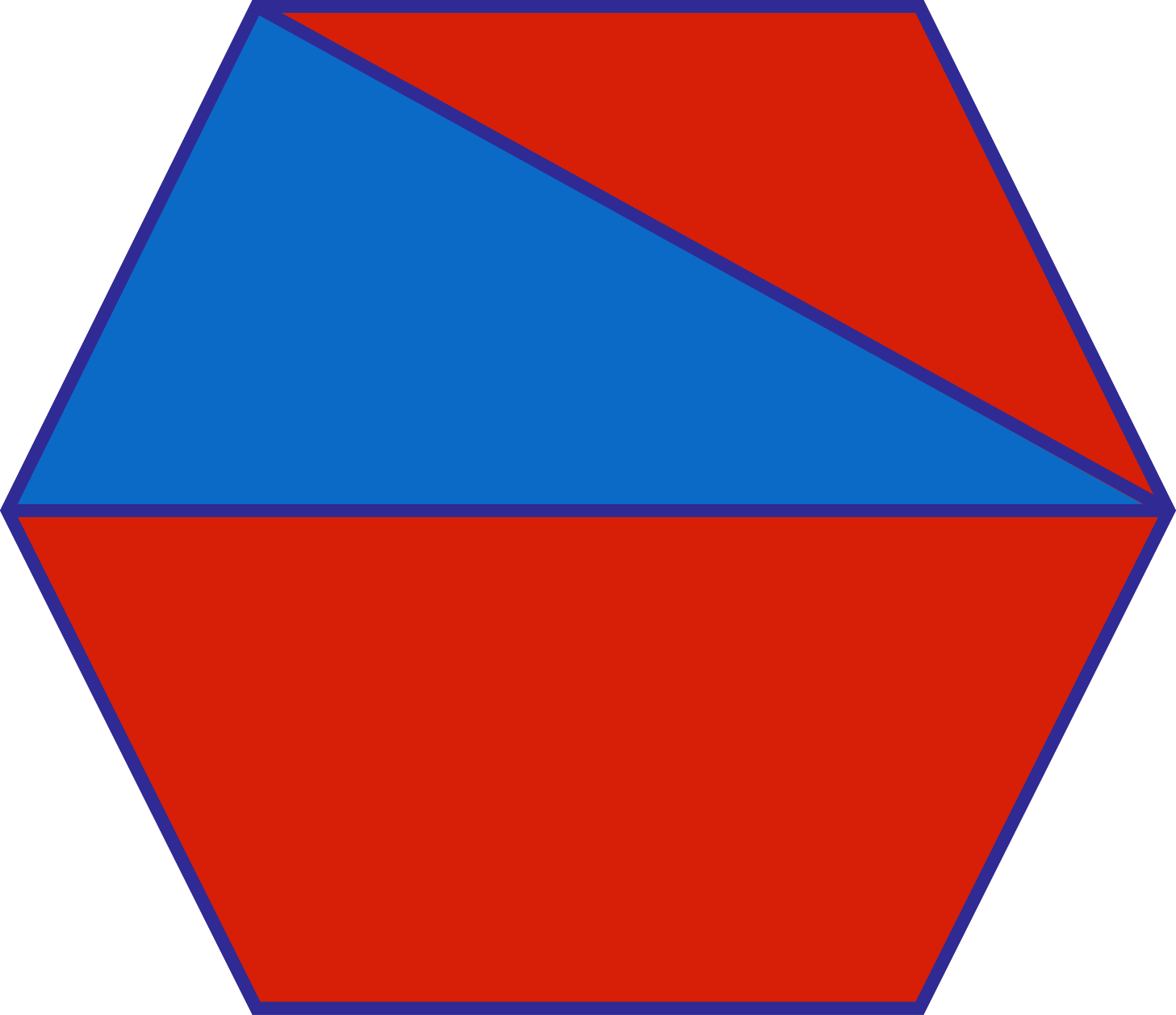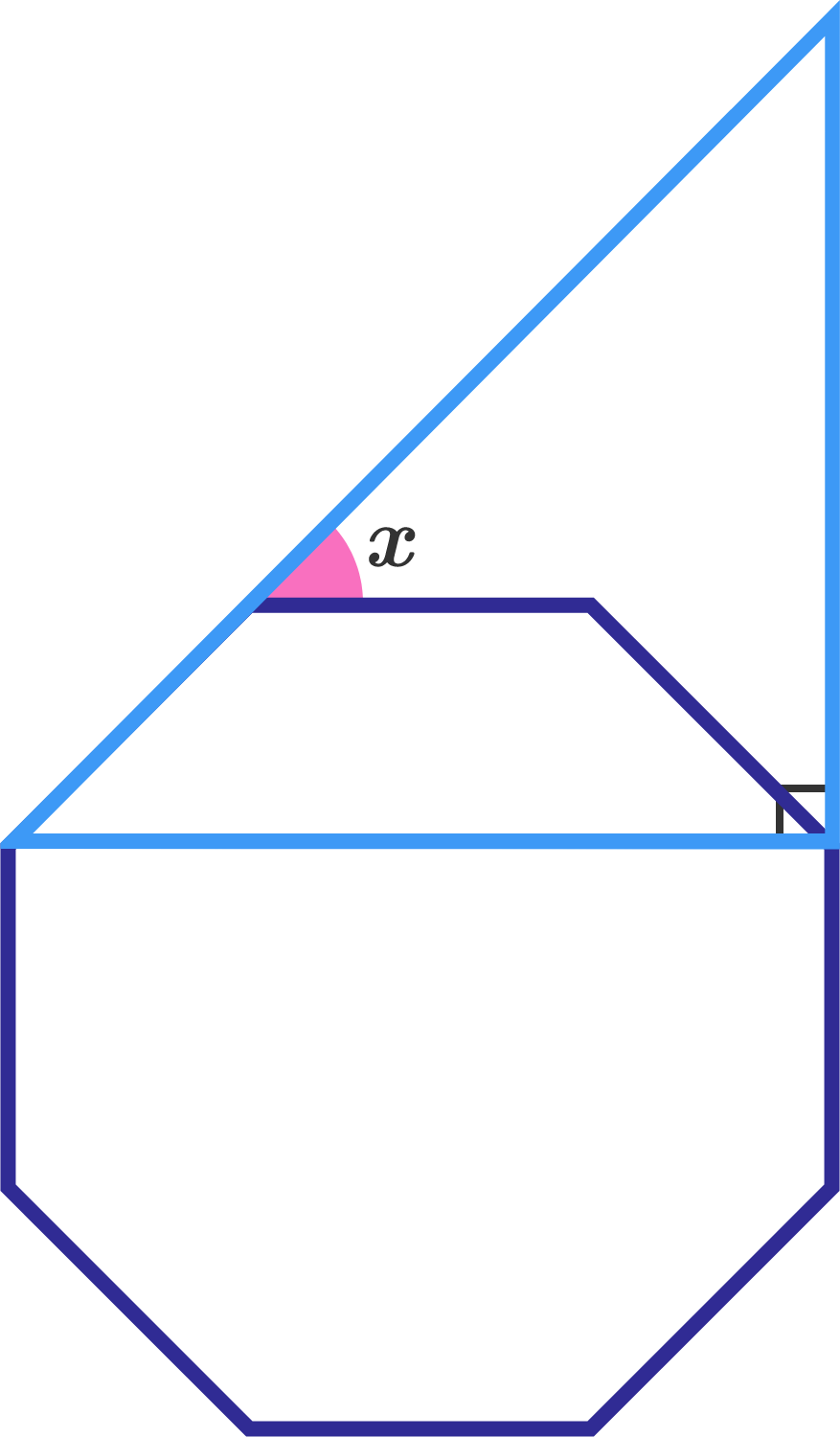Geometry

# Regular Polygons: Level 1 ChallengesRegular $n$-gon Internal Angle Sum 3 180 4 360 5 540 6 720

Given the pattern seen in this table, which of the following accurately represents the internal angle sum in terms of $n$?If $FGHIJ$ is a regular pentagon, find $\angle A + \angle B + \angle C + \angle D + \angle E \ .$

Details: A regular pentagon is a pentagon with 5 sides of equal length and 5 corner angles of equal measure.What is the area of the red region if the area of the blue region is 5?


Note: The hexagon is regular.

Which of the following is the ratio of the measure of an interior angle of a 24-sided regular polygon to that of a 12-sided regular polygon?Consider that in the above image the triangle is right angled and the octagon is regular. Then find $x$ in degrees.

×